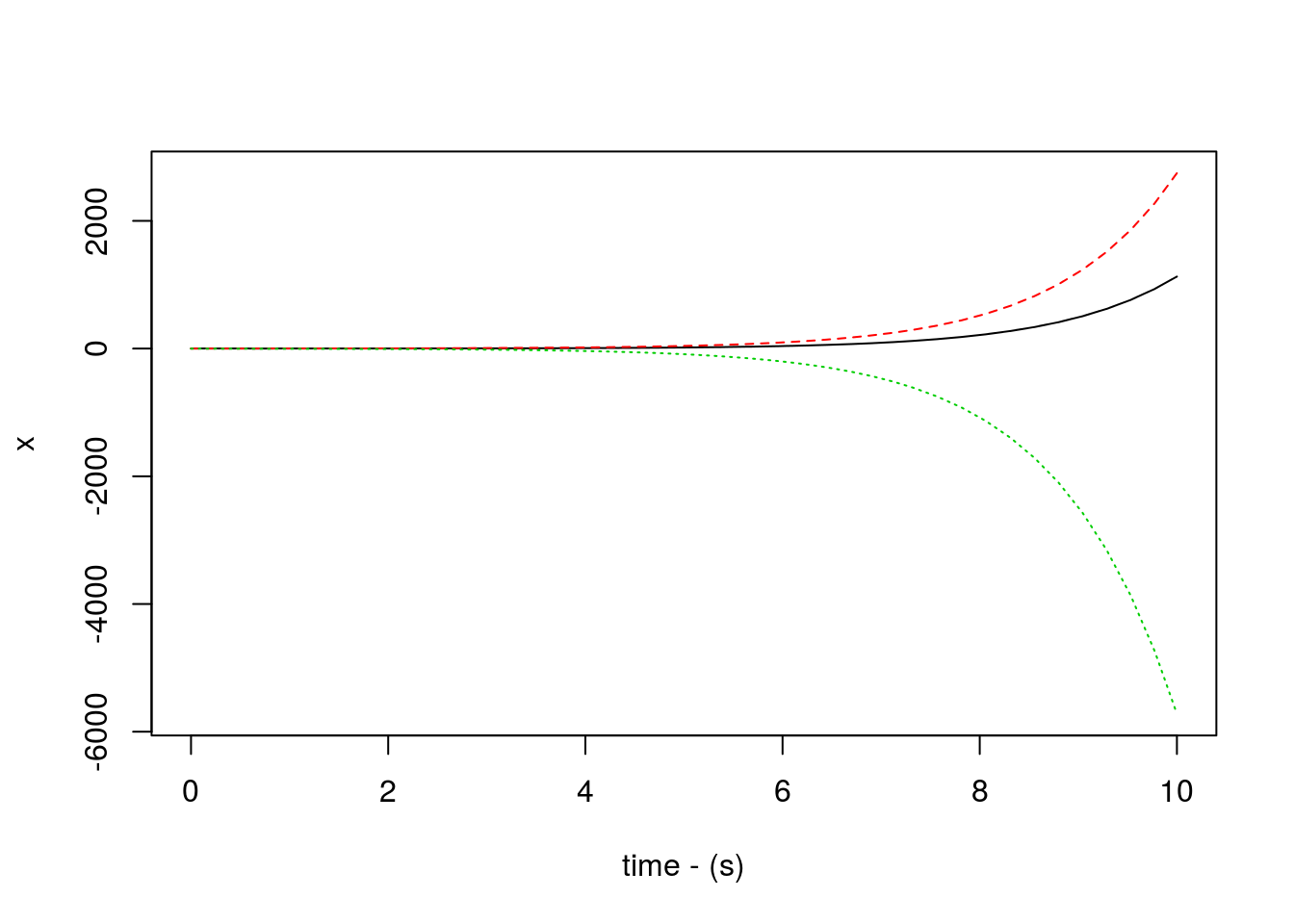Note: If you wish to replicate the R code below, then you will need to copy and paste the following commands in R first (to make sure you have all the packages and their dependencies):

install.packages("install.load")
# install the install.load package

# you will need to have GNU Octave (check the RcppOctave CRAN page for more details) installed on your system, then you can use the code below:

install.load::install_library("ramify", "RcppOctave")
# install and/or load the packages and their dependencies, including the extra system dependencies (this process may take a while depending on the number of dependencies)

install.packages("import")
# install the import package

import::from(pracma, ode45)
# import ode45 from the pracma package

This document was created with rmarkdown 1.3 using the following:

• R 3.3.2 (2016-10-31)
• ramify 0.3.3
• RcppOctave 0.18.1
• import 1.1.0
• pracma 1.9.5

# Example 1

library(ramify)

import::from(pracma, ode45)
# import ode45 from the pracma package

# Source 1 begins
source("https://raw.githubusercontent.com/ggrothendieck/gsubfn/master/R/list.R")

GRT <- function(t, x) {
z1 <- x[1, 1]
z2 <- x[2, 1]
z3 <- x[3, 1]
X <- matrix(data = c(z1, z2, z3), nrow = 3, ncol = 1)
xd <- A %*% X + B * exp(-t) * sin(400 * t)
return(xd)
}

A <- -mat("2, 3, 2; 1, 5, 3; 2, 3, 1")
B <- mat("1; 3; 2")
ts <- 0
tf <- 10
X0 <- c(1, 0, -1)

list[t, x] <- ode45(GRT, ts, tf, X0, atol = 1e-06, hmax = 1)

matplot(t, x, xlab = "time - (s)", ylab = "x", type = "l")# Source 1 ends

The following code uses RcppOctave. The plots will only show up if you have installed RcppOctave and then run the code.

library(RcppOctave)

o_source(text = "
function xd = GRT(t, x)
global A B
z1 = x(1, 1); z2 = x(2, 1); z3 = x(3, 1); X = [z1; z2; z3];
xd = A * X + B * exp(-t) * sin(400 * t);
endfunction % only needed for GNU Octave

global A B
A = -[2, 3, 2; 1, 5, 3; 2, 3, 1];
B = [1; 3; 2];
ts = 0.0;
tf = 10;
T = [ts, tf]; X0 = [1, 0, -1];
[t, x] = ode45(@GRT, T, X0);
P = [t, x];
plot(t, x)
xlabel('time - (s)');
ylabel('x');
")

# Example 2

For the following state equations plot y versus x for the following initial conditions: [1, 1, 1]’, [1, 2, 1]’, [1, 5, 1]’, [1, 10, 1]’, [10, 2, 1]’, [10, 5, 1]’, [10, 10, 1]’

$\dot{x} = −y − z$ $\dot{y} = x + ay$ $\dot{z} = b + z(x − c)$

Where a = b = 0.2 and c = 5.7

import::from(pracma, ode45)
# import ode45 from the pracma package

source("https://raw.githubusercontent.com/ggrothendieck/gsubfn/master/R/list.R")

dydx2 <- function(t, y) {
a <- 0.2
b <- 0.2
c <- 5.7

X1 <- -y - y
X2 <- y + a * y
X3 <- b + (y * (y - c))

dy <- matrix(data = c(X1, X2, X3), nrow = 3, ncol = 1)

return(dy)
}

ts <- 0
tf <- 10
X0 <- c(1, 0, -1)

ts <- 0
tf <- 50

X0 <- c(1, 1, 1)
list[t, y] <- ode45(dydx2, ts, tf, X0, atol = 1e-06, hmax = 1)
matplot(y[, 1], y[, 2], xlab = "x", ylab = "y", type = "l", main = "x-y Phase Plane Plot",
xlim = c(-18, 18), ylim = c(-21, 16))

X0 <- c(1, 2, 1)
list[t, y] <- ode45(dydx2, ts, tf, X0, atol = 1e-06, hmax = 1)
matplot(y[, 1], y[, 2], type = "l", add = TRUE)

X0 <- c(1, 5, 1)
list[t, y] <- ode45(dydx2, ts, tf, X0, atol = 1e-06, hmax = 1)
matplot(y[, 1], y[, 2], type = "l", add = TRUE)

X0 <- c(1, 10, 1)
list[t, y] <- ode45(dydx2, ts, tf, X0, atol = 1e-06, hmax = 1)
matplot(y[, 1], y[, 2], type = "l", add = TRUE)

X0 <- c(10, 2, 1)
list[t, y] <- ode45(dydx2, ts, tf, X0, atol = 1e-06, hmax = 1)
matplot(y[, 1], y[, 2], type = "l", add = TRUE)

X0 <- c(10, 5, 1)
list[t, y] <- ode45(dydx2, ts, tf, X0, atol = 1e-06, hmax = 1)
matplot(y[, 1], y[, 2], type = "l", add = TRUE)

X0 <- c(10, 10, 1)
list[t, y] <- ode45(dydx2, ts, tf, X0, atol = 1e-06, hmax = 1)
matplot(y[, 1], y[, 2], type = "l", add = TRUE)